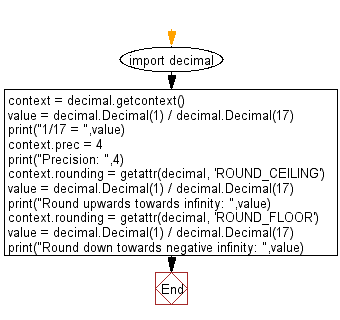﻿ Python Math: Round a specified number upwards towards infinity and down towards negative infinity - w3resource# Python Math: Round a specified number upwards towards infinity and down towards negative infinity

## Python Math: Exercise-41 with Solution

Write a Python program to round a specified number upwards towards infinity and down towards negative infinity of precision 4.

Sample Solution:-

Python Code:

``````import decimal
context = decimal.getcontext()
value = decimal.Decimal(1) / decimal.Decimal(17)
print("1/17 = ",value)
context.prec = 4
print("Precision: ",4)

context.rounding = getattr(decimal, 'ROUND_CEILING')
value = decimal.Decimal(1) / decimal.Decimal(17)
print("Round upwards towards infinity: ",value)

context.rounding = getattr(decimal, 'ROUND_FLOOR')
value = decimal.Decimal(1) / decimal.Decimal(17)
print("Round down towards negative infinity: ",value)
```
```

Sample Output:

```1/17 =  0.05882352941176470588235294118
Precision:  4
Round upwards towards infinity:  0.05883
Round down towards negative infinity:  0.05882
```

Flowchart:Python Code Editor:

Have another way to solve this solution? Contribute your code (and comments) through Disqus.

What is the difficulty level of this exercise?

Test your Programming skills with w3resource's quiz.

﻿

```>>> students = [{'name': 'John', 'score': 98}, {'name': 'Mike', 'score': 94}, {'name': 'Jennifer', 'score': 99}]